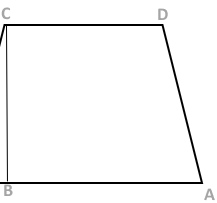Courses
Courses for Kids
Free study material
Free LIVE classes
MoreLIVE
Join Vedantu’s FREE Mastercalss

# ABCD is a trapezium such that AB is parallel to CD and CB is perpendicular to them. If $\angle ADB = \theta$, BC=p and CD=q, show that: $AB = \dfrac{{({p^2} + {q^2})\sin \theta }}{{p\cos \theta + q\sin \theta }}$Verified
Hint: $\vartriangle BCD$ is a right-angled triangle so we can use Pythagoras theorem. Also the sum of all the angles of the triangle is $\pi$.
From the right-angle triangle $\vartriangle BCD$, we get, $BD = \sqrt {{p^2} + {q^2}}$ . Let $\angle ADB = \angle BDC = \alpha$ then $\angle DAB = \pi - (\alpha - \theta )$ and $\tan \alpha = \dfrac{p}{q}$. Now, from $\vartriangle ABD$, we have
$\dfrac{{AB}}{{\sin \theta }} = \dfrac{{BD}}{{\sin [\pi - (\theta + \alpha )]}} = \dfrac{{BD}}{{\sin (\theta + \alpha )}} \\ \therefore AB = \dfrac{{BD\sin \theta }}{{\sin (\theta + \alpha )}} = \dfrac{{B{D^2}\sin \theta }}{{BD\sin (\theta + \alpha )}} \\ = \dfrac{{B{D^2}\sin \theta }}{{BD\sin \theta \cos \alpha + BD\cos \theta \sin \alpha }} \\ = \dfrac{{({p^2} + {q^2})\sin \theta }}{{q\sin \theta + p\cos \theta }} \\$